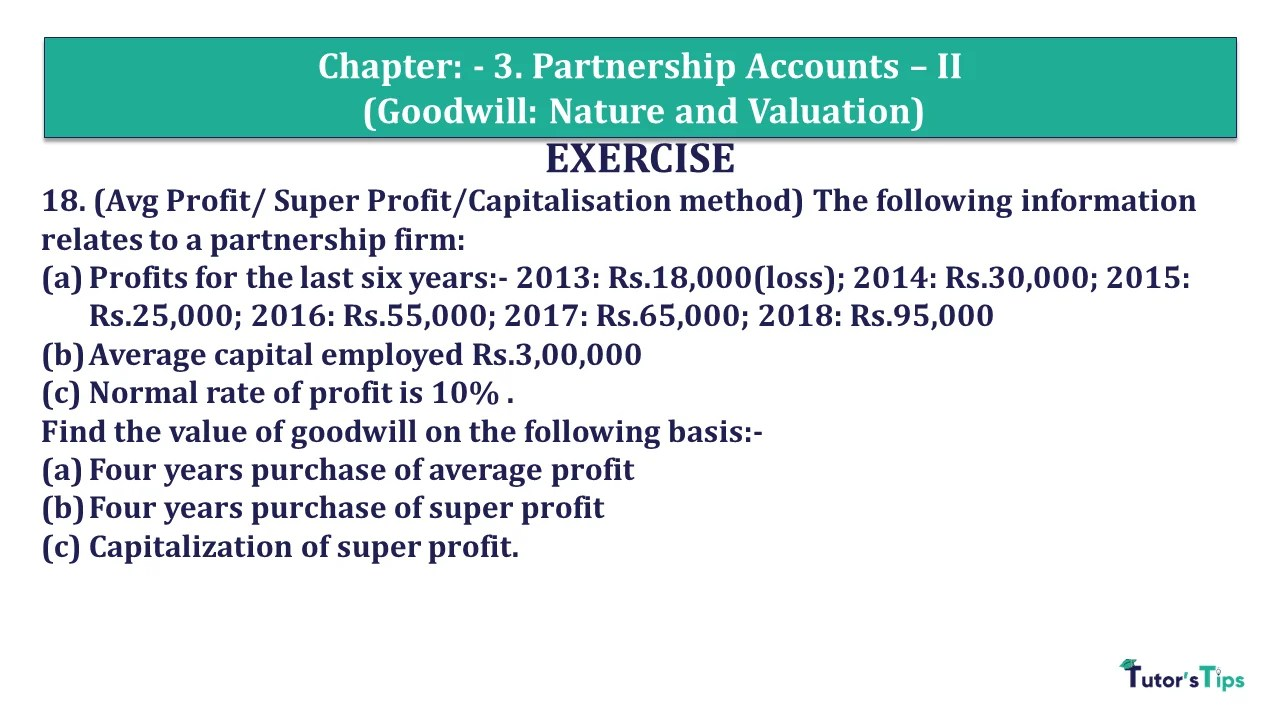# Question 19 Chapter 3 of +2 Part-1 – USHA Publication 12 Class Part – 1Q-19 - CH-3 - Usha +2 Book 2018 - Solution

Question 19 Chapter 3 of +2-Part-1

18. (Avg Profit/ Super Profit/Capitalisation method) The following information relates to a partnership firm:

a. Profits for the last six years:- 2013: Rs.18,000(loss); 2014: Rs.30,000; 2015: Rs.25,000; 2016: Rs.55,000; 2017: Rs.65,000; 2018: Rs.95,000

b. Average capital employed Rs.3,00,000

c. Normal rate of profit is 10%
Find the value of goodwill on the following basis:

a. Four years purchase of average profit

b. Four years purchase of super profit

c. Capitalization of super profit.

## The solution of Question 19 Chapter 3 of +2 Part-1: –

i) Calculation of Goodwill with average Profit

 Number of years’ purchase = 3 Goodwill = Average Profit  X Number of years’ purchase = 42,000 X 4 Goodwill = 1,68,000

ii) Four years purchase of super profit.

 Number of years’ purchase = 4 Goodwill = Super Profit  X Number of years’ purchase = 12,000 X 4 Goodwill = 48,000

iii) On the basis of Capitalisation of Super Profit.

 Goodwill = Super Profit X 100 Normal Rate of Return = 12,000 X 100 10 = 1,20,000

Working Notes: –

*1 Calculation of Average Profit

 Average Profit = Profit for past given years Number of years = (-18,000) + 30,000+ 25,000 + 55,000 + 65,000+ 95,000 6 = 2,52,000 6 = 42,000

*2 Calculation of Super Profit

 Normal Profit = Capital Employed X Normal Rate of Return 100 = 3,00,000 X 10 100 = 30,000

 Super Profit = Actual Profit  – Normal Profit = 42,000 – 30,000 = 12,000